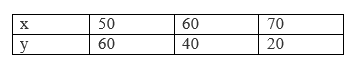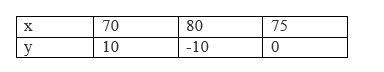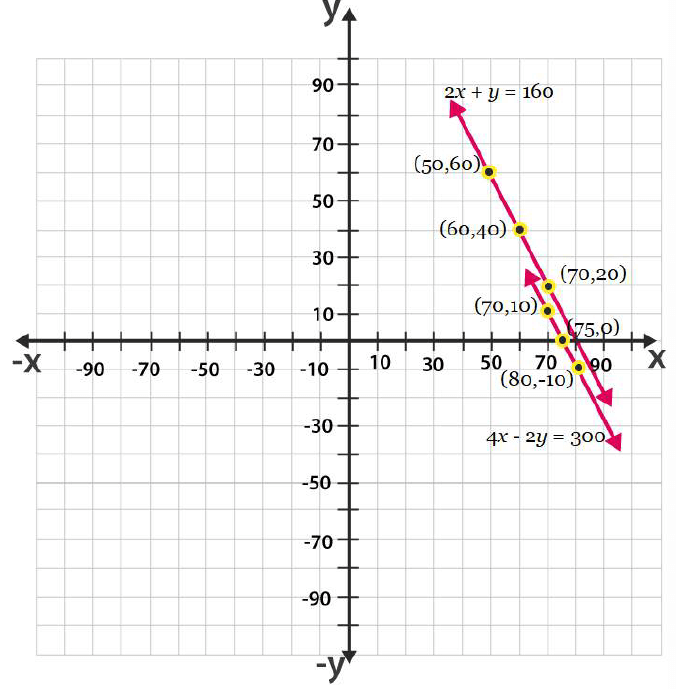Guru

# The cost of 2 kg of apples and 1kg of grapes on a day was found to be Rs.160. After a month, the cost of 4 kg of apples and 2 kg of grapes is Rs.300. Represent the situation algebraically and geometrically. Q.3

• 1

Tell me the answer of the question of exercise 3.1 question no.3. I don’t know how to solve this question. Please help me for finding the answer of this question The cost of 2 kg of apples and 1kg of grapes on a day was found to be Rs.160. After a month, the cost of 4 kg of apples and 2 kg of grapes is Rs.300. Represent the situation algebraically and geometrically.

Share

1. Let the cost of 1 kg of apples be ‘Rs. x’

And, cost of 1 kg of grapes be ‘Rs. y’

According to the question, the algebraic representation is

2x+y = 160

And 4x+2y = 300

For, 2x+y = 160 or y = 160−2x, the solution table is;For 4x+2y = 300 or y = (300-4x)/2, the solution table is;The graphical representation is as follows;• 0